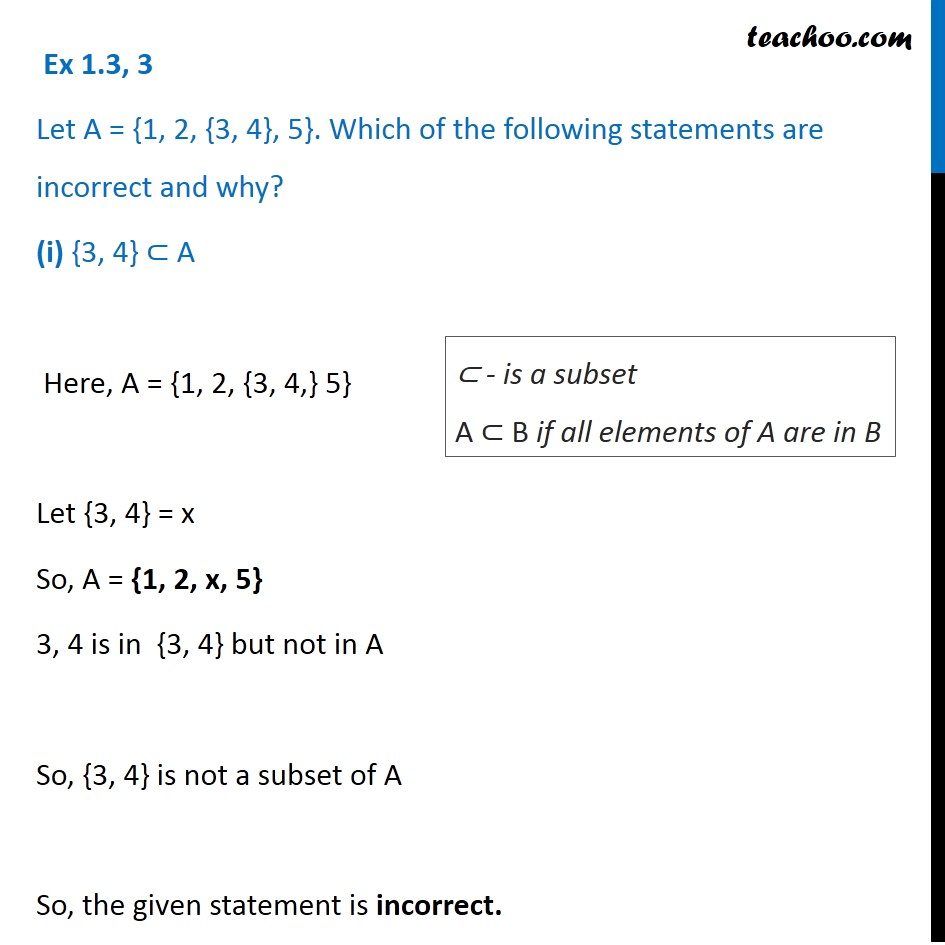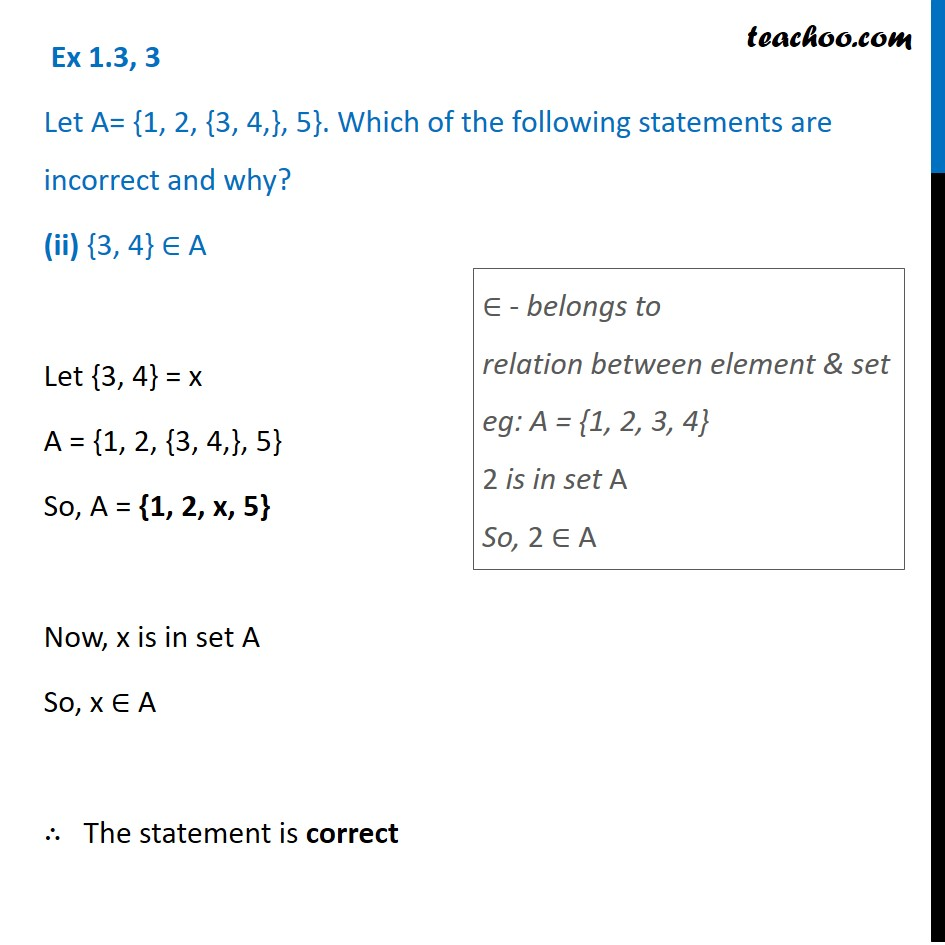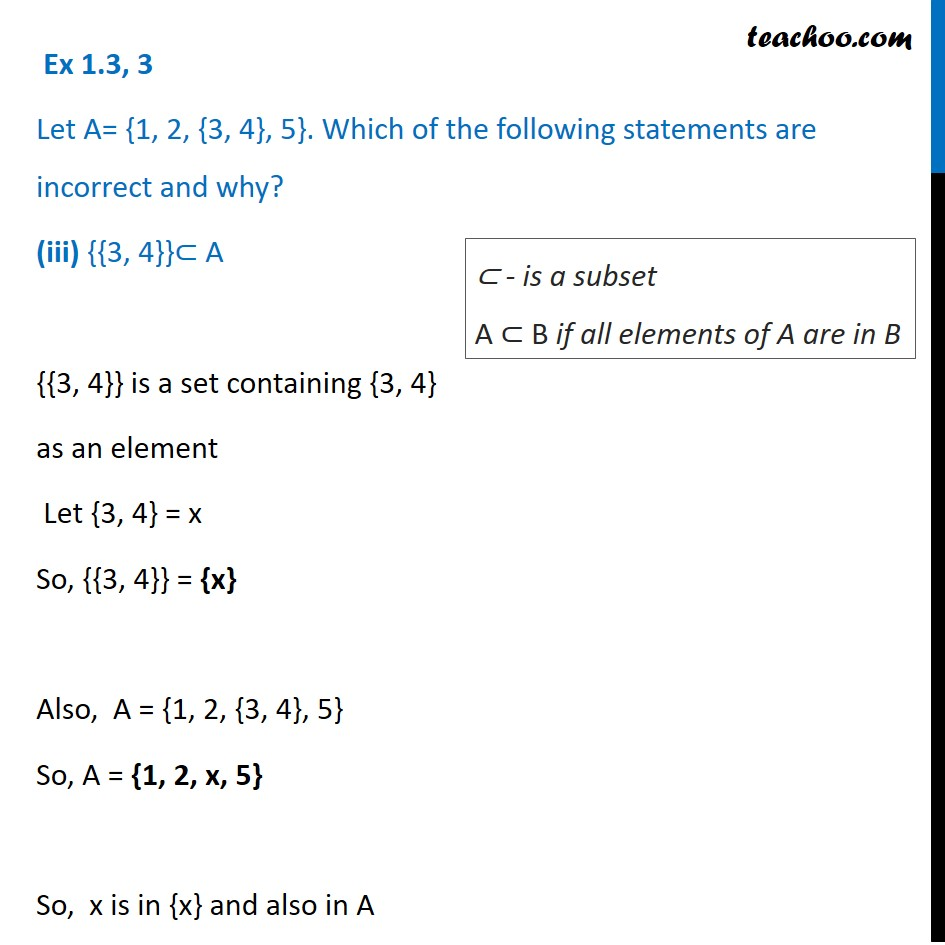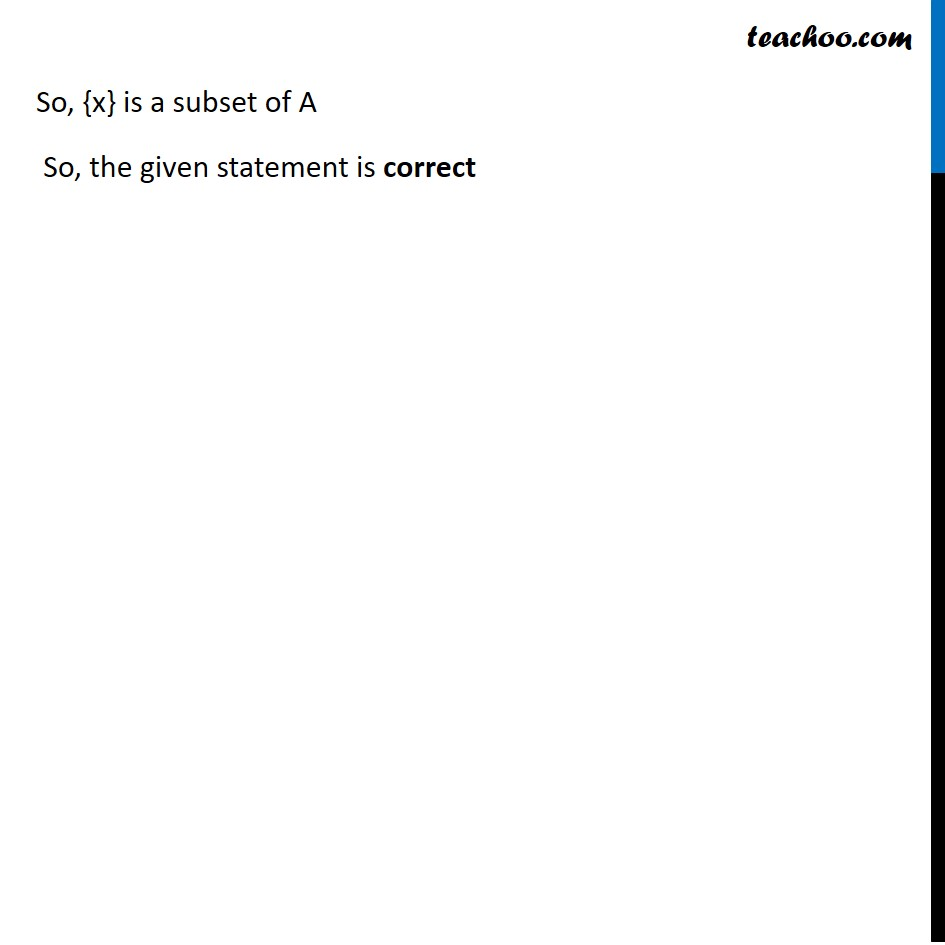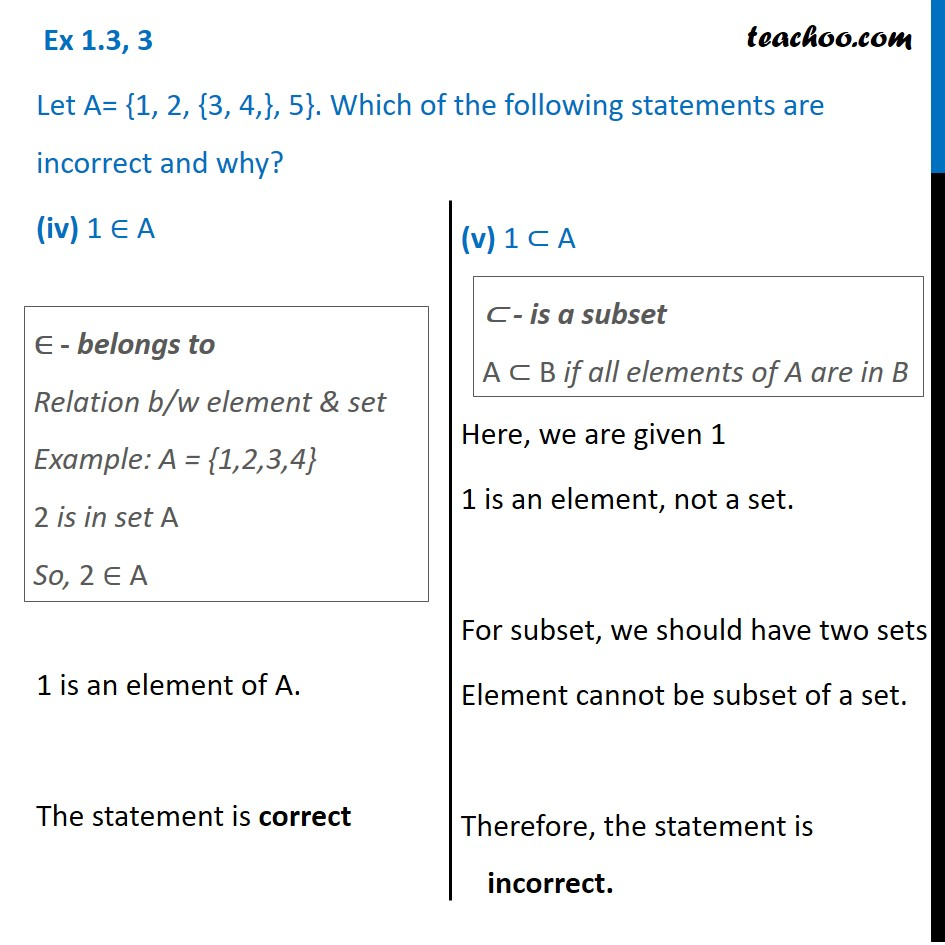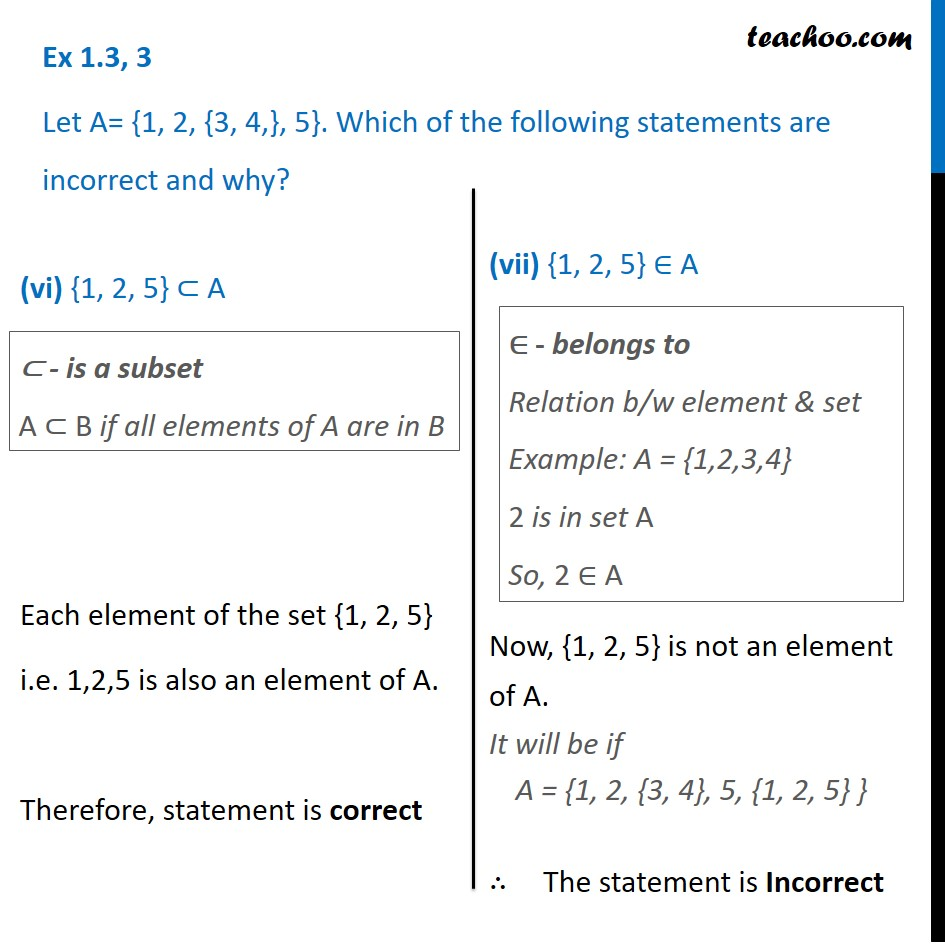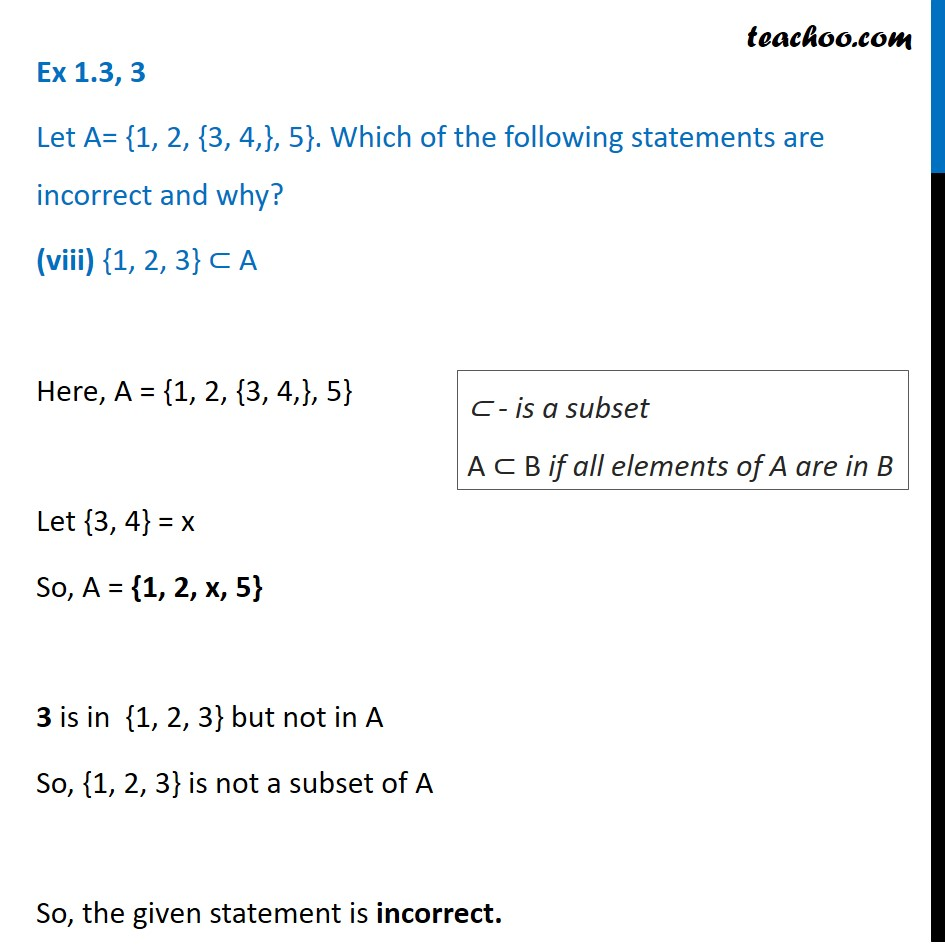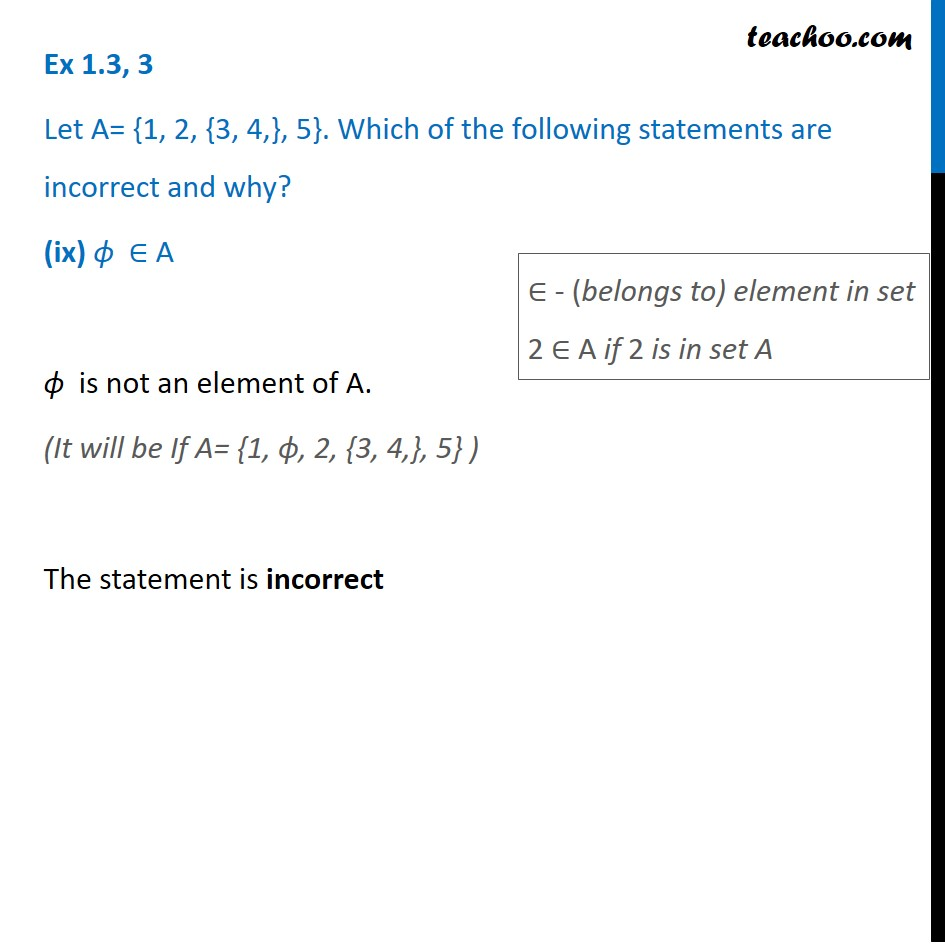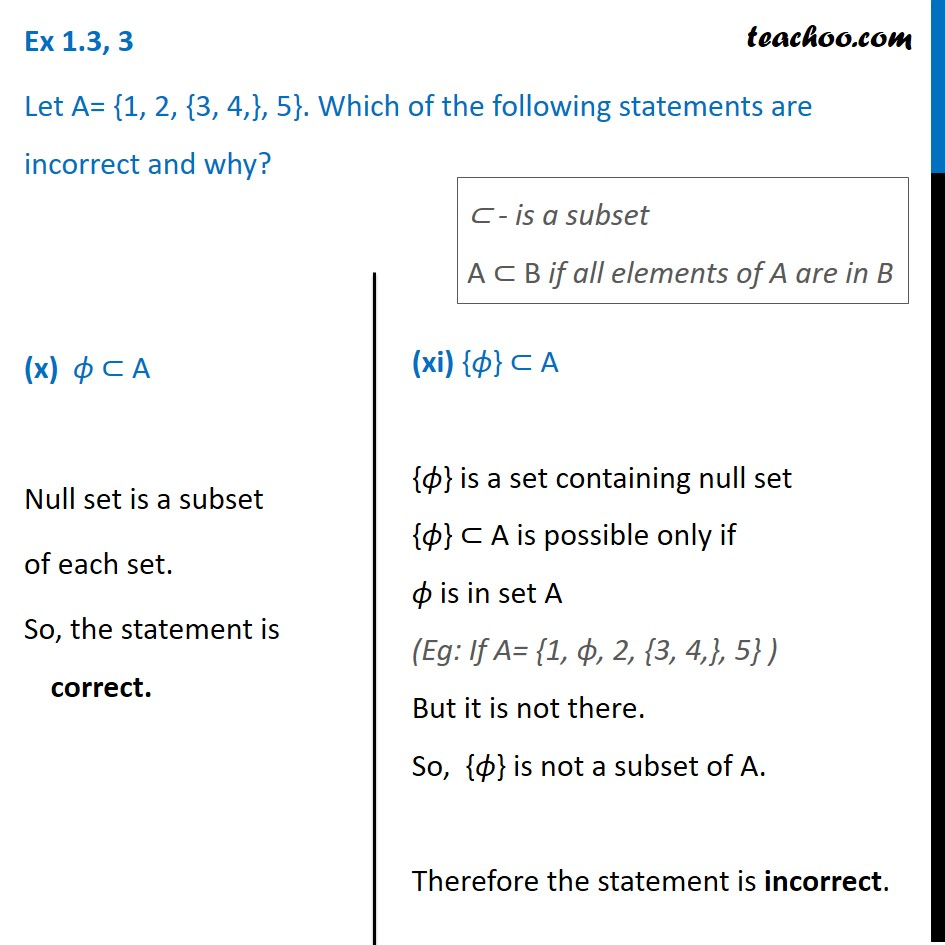1. Chapter 1 Class 11 Sets
2. Concept wise
3. Subset

Transcript

Ex 1.3, 3 Let A = {1, 2, {3, 4}, 5}. Which of the following statements are incorrect and why? (i) {3, 4} ⊂ A Here, A = {1, 2, {3, 4,} 5} Let {3, 4} = x So, A = {1, 2, x, 5} 3, 4 is in {3, 4} but not in A So, {3, 4} is not a subset of A So, the given statement is incorrect. Ex 1.3, 3 Let A = {1, 2, {3, 4}, 5}. Which of the following statements are incorrect and why? (i) {3, 4} ⊂ A Here, A = {1, 2, {3, 4,} 5} Let {3, 4} = x So, A = {1, 2, x, 5} 3, 4 is in {3, 4} but not in A So, {3, 4} is not a subset of A So, the given statement is incorrect. ⊂ - is a subset A ⊂ B if all elements of A are in B Ex 1.3, 3 Let A= {1, 2, {3, 4,}, 5}. Which of the following statements are incorrect and why? (ii) {3, 4} ∈ A Let {3, 4} = x A = {1, 2, {3, 4,}, 5} So, A = {1, 2, x, 5} Now, x is in set A So, x ∈ A ∴ The statement is correct ∈ - belongs to relation between element & set eg: A = {1, 2, 3, 4} 2 is in set A So, 2 ∈ A Ex 1.3, 3 Let A= {1, 2, {3, 4}, 5}. Which of the following statements are incorrect and why? (iii) {{3, 4}}⊂ A {{3, 4}} is a set containing {3, 4} as an element Let {3, 4} = x So, {{3, 4}} = {x} Also, A = {1, 2, {3, 4}, 5} So, A = {1, 2, x, 5} So, x is in {x} and also in A ⊂ - is a subset A ⊂ B if all elements of A are in B So, {x} is a subset of A So, the given statement is correct Ex 1.3, 3 Let A= {1, 2, {3, 4,}, 5}. Which of the following statements are incorrect and why? (iv) 1 ∈ A 1 is an element of A. The statement is correct ∈ - belongs to Relation b/w element & set Example: A = {1,2,3,4} 2 is in set A So, 2 ∈ A ⊂ - is a subset A ⊂ B if all elements of A are in B (v) 1 ⊂ A Here, we are given 1 1 is an element, not a set. For subset, we should have two sets Element cannot be subset of a set. Therefore, the statement is incorrect. Ex 1.3, 3 Let A= {1, 2, {3, 4,}, 5}. Which of the following statements are incorrect and why? (vi) {1, 2, 5} ⊂ A Each element of the set {1, 2, 5} i.e. 1,2,5 is also an element of A. Therefore, statement is correct ⊂ - is a subset A ⊂ B if all elements of A are in B ∈ - belongs to Relation b/w element & set Example: A = {1,2,3,4} 2 is in set A So, 2 ∈ A (vii) {1, 2, 5} ∈ A Now, {1, 2, 5} is not an element of A. It will be if A = {1, 2, {3, 4}, 5, {1, 2, 5} } ∴ The statement is Incorrect Ex 1.3, 3 Let A= {1, 2, {3, 4,}, 5}. Which of the following statements are incorrect and why? (viii) {1, 2, 3} ⊂ A Here, A = {1, 2, {3, 4,}, 5} Let {3, 4} = x So, A = {1, 2, x, 5} 3 is in {1, 2, 3} but not in A So, {1, 2, 3} is not a subset of A So, the given statement is incorrect. ⊂ - is a subset A ⊂ B if all elements of A are in B Ex 1.3, 3 Let A= {1, 2, {3, 4,}, 5}. Which of the following statements are incorrect and why? (ix) ϕ ∈ A ϕ is not an element of A. (It will be If A= {1, ϕ, 2, {3, 4,}, 5} ) The statement is incorrect Ex 1.3, 3 Let A= {1, 2, {3, 4,}, 5}. Which of the following statements are incorrect and why? (ix) ϕ ∈ A ϕ is not an element of A. (It will be If A= {1, ϕ, 2, {3, 4,}, 5} ) The statement is incorrect ∈ - (belongs to) element in set 2 ∈ A if 2 is in set A Ex 1.3, 3 Let A= {1, 2, {3, 4,}, 5}. Which of the following statements are incorrect and why? (x) ϕ ⊂ A Null set is a subset of each set. So, the statement is correct. ⊂ - is a subset A ⊂ B if all elements of A are in B (xi) {ϕ} ⊂ A {ϕ} is a set containing null set {ϕ} ⊂ A is possible only if ϕ is in set A (Eg: If A= {1, ϕ, 2, {3, 4,}, 5} ) But it is not there. So, {ϕ} is not a subset of A. Therefore the statement is incorrect.

Subset